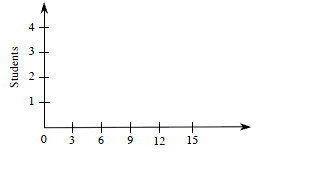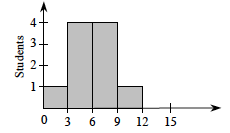### Home > MC1 > Chapter 1 > Lesson 1.3.1 > Problem1-103

1-103.

Given the data in the table below, copy and complete the histogram at right to represent families of various sizes. Remember that data that falls on a tick mark (that is, $3$, $6$, $9$, ...) goes in the column to the right of that mark.

Student

# of family members

LaTrese

$4$

James

$8$

Phu

$7$

Byron

$3$

Evan

$2$

Diamond

$11$

Jackie

$5$

Antonio

$5$

Shinna

$6$

Ryan

$8$Look at problem 1-100 in your book to get you started on this histogram. Make sure to try creating your own graph before checking the answer.

Begin by counting how many students have families with less than $3$ people, with $3$ to $5$ people, with $6$ to $8$ people, and with $9$ to $11$ people (since none have more than $12$).

Families with less than $3$ people: $1$
Families with $3$-$5$ people: $4$
Families with $6$-$8$ people: $4$
Families with $9$-$11$ people: $1$

In what range do the most number of family members fall? Remember to answer all of the questions.